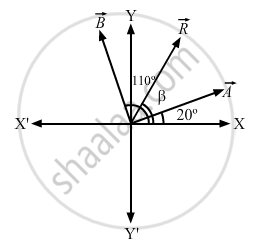Department of Pre-University Education, KarnatakaPUC Karnataka Science Class 11

# A Vector → a Makes an Angle of 20° and → B Makes an Angle of 110° with the X-axis. the Magnitudes of These Vectors Are 3 M and 4 M Respectively. Find the Resultant. - Physics

A vector $\vec{A}$ makes an angle of 20° and $\vec{B}$ makes an angle of 110° with the X-axis. The magnitudes of these vectors are 3 m and 4 m respectively. Find the resultant.

#### SolutionFrom the above figure, we have:
Angle between $\vec{A}$ and $\vec{B}$ = 110° − 20° = 90° $\left| \vec{A} \right| = 3 \text{ m } \text{ and } \left| \vec{B} \right| = \text{ 4 }m$

Magnitude of the resultant vector is given by

$R = \sqrt{A^2 + B^2 + 2AB \cos \theta}$

$= \sqrt{3^2 + 4^2 + 2 \times 3 \times 4 \times \cos 90^\circ}$

$= \text{ 5 }m$

Let β be the angle between $\vec{R} \text { and } \vec{A}$.

$\beta = \tan^{- 1} \left( \frac{A \sin 90^\circ}{A + B \cos 90^\circ} \right)$

$= \tan^{- 1} \left( \frac{4 \sin 90^\circ}{3 + 4 \cos 90^\circ} \right)$

$= \tan^{- 1} \frac{4}{3}$

$= \tan^{- 1} \left( 1 . 333 \right)$

$= 53^\circ$

Now, angle made by the resultant vector with the X-axis = 53° + 20° = 73°
∴ The resultant $\vec{R}$ is 5 m and it makes an angle of 73° with the x-axis.

Concept: What is Physics?
Is there an error in this question or solution?

#### APPEARS IN

HC Verma Class 11, Class 12 Concepts of Physics Vol. 1
Chapter 2 Physics and Mathematics
Exercise | Q 1 | Page 29
Share